# garage.torch.algos¶

PyTorch algorithms.

class BC(env_spec, learner, *, batch_size, source=None, policy_optimizer=torch.optim.Adam, policy_lr=_Default(0.001), loss='log_prob', minibatches_per_epoch=16, name='BC')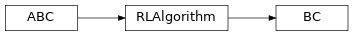Behavioral Cloning.

Based on Model-Free Imitation Learning with Policy Optimization:

https://arxiv.org/abs/1605.08478

Parameters
• env_spec (EnvSpec) – Specification of environment.

• learner (garage.torch.Policy) – Policy to train.

• batch_size (int) – Size of optimization batch.

• source (Policy or Generator[TimeStepBatch]) – Expert to clone. If a policy is passed, will set .policy to source and use the trainer to sample from the policy.

• policy_optimizer (torch.optim.Optimizer) – Optimizer to be used to optimize the policy.

• policy_lr (float) – Learning rate of the policy optimizer.

• loss (str) – Which loss function to use. Must be either ‘log_prob’ or ‘mse’. If set to ‘log_prob’ (the default), learner must be a garage.torch.StochasticPolicy.

• minibatches_per_epoch (int) – Number of minibatches per epoch.

• name (str) – Name to use for logging.

Raises

ValueError – If learner is not a garage.torch.StochasticPolicy and loss is ‘log_prob’.

train(self, trainer)

Obtain samplers and start actual training for each epoch.

Parameters

trainer (Trainer) – Experiment trainer, for services such as snapshotting and sampler control.

class DDPG(env_spec, policy, qf, replay_buffer, *, steps_per_epoch=20, n_train_steps=50, max_episode_length_eval=None, buffer_batch_size=64, min_buffer_size=int(10000.0), exploration_policy=None, target_update_tau=0.01, discount=0.99, policy_weight_decay=0, qf_weight_decay=0, policy_optimizer=torch.optim.Adam, qf_optimizer=torch.optim.Adam, policy_lr=_Default(0.0001), qf_lr=_Default(0.001), clip_pos_returns=False, clip_return=np.inf, max_action=None, reward_scale=1.0)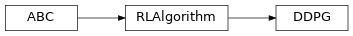A DDPG model implemented with PyTorch.

DDPG, also known as Deep Deterministic Policy Gradient, uses actor-critic method to optimize the policy and Q-function prediction. It uses a supervised method to update the critic network and policy gradient to update the actor network. And there are exploration strategy, replay buffer and target networks involved to stabilize the training process.

Parameters
• env_spec (EnvSpec) – Environment specification.

• policy (garage.torch.policies.Policy) – Policy.

• qf (object) – Q-value network.

• replay_buffer (ReplayBuffer) – Replay buffer.

• steps_per_epoch (int) – Number of train_once calls per epoch.

• n_train_steps (int) – Training steps.

• buffer_batch_size (int) – Batch size of replay buffer.

• min_buffer_size (int) – The minimum buffer size for replay buffer.

• exploration_policy (garage.np.exploration_policies.ExplorationPolicy) – # noqa: E501 Exploration strategy.

• target_update_tau (float) – Interpolation parameter for doing the soft target update.

• max_episode_length_eval (int or None) – Maximum length of episodes used for off-policy evaluation. If None, defaults to env_spec.max_episode_length.

• discount (float) – Discount factor for the cumulative return.

• policy_weight_decay (float) – L2 weight decay factor for parameters of the policy network.

• qf_weight_decay (float) – L2 weight decay factor for parameters of the q value network.

• policy_optimizer (Union[type, tuple[type, dict]]) – Type of optimizer for training policy network. This can be an optimizer type such as torch.optim.Adam or a tuple of type and dictionary, where dictionary contains arguments to initialize the optimizer e.g. (torch.optim.Adam, {‘lr’ : 1e-3}).

• qf_optimizer (Union[type, tuple[type, dict]]) – Type of optimizer for training Q-value network. This can be an optimizer type such as torch.optim.Adam or a tuple of type and dictionary, where dictionary contains arguments to initialize the optimizer e.g. (torch.optim.Adam, {‘lr’ : 1e-3}).

• policy_lr (float) – Learning rate for policy network parameters.

• qf_lr (float) – Learning rate for Q-value network parameters.

• clip_pos_returns (bool) – Whether or not clip positive returns.

• max_action (float) – Maximum action magnitude.

• reward_scale (float) – Reward scale.

train(self, trainer)

Obtain samplers and start actual training for each epoch.

Parameters

trainer (Trainer) – Experiment trainer.

Returns

The average return in last epoch cycle.

Return type

float

train_once(self, itr, episodes)

Perform one iteration of training.

Parameters
• itr (int) – Iteration number.

• episodes (EpisodeBatch) – Batch of episodes.

optimize_policy(self, samples_data)

Perform algorithm optimizing.

Parameters

samples_data (dict) – Processed batch data.

Returns

Loss of action predicted by the policy network. qval_loss: Loss of Q-value predicted by the Q-network. ys: y_s. qval: Q-value predicted by the Q-network.

Return type

action_loss

update_target(self)

Update parameters in the target policy and Q-value network.

class VPG(env_spec, policy, value_function, policy_optimizer=None, vf_optimizer=None, num_train_per_epoch=1, discount=0.99, gae_lambda=1, center_adv=True, positive_adv=False, policy_ent_coeff=0.0, use_softplus_entropy=False, stop_entropy_gradient=False, entropy_method='no_entropy')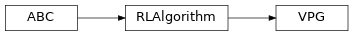VPG, also known as Reinforce, trains stochastic policy in an on-policy way.

Parameters
• env_spec (EnvSpec) – Environment specification.

• policy (garage.torch.policies.Policy) – Policy.

• value_function (garage.torch.value_functions.ValueFunction) – The value function.

• policy_optimizer (garage.torch.optimizer.OptimizerWrapper) – Optimizer for policy.

• vf_optimizer (garage.torch.optimizer.OptimizerWrapper) – Optimizer for value function.

• num_train_per_epoch (int) – Number of train_once calls per epoch.

• discount (float) – Discount.

• gae_lambda (float) – Lambda used for generalized advantage estimation.

• center_adv (bool) – Whether to rescale the advantages so that they have mean 0 and standard deviation 1.

• positive_adv (bool) – Whether to shift the advantages so that they are always positive. When used in conjunction with center_adv the advantages will be standardized before shifting.

• policy_ent_coeff (float) – The coefficient of the policy entropy. Setting it to zero would mean no entropy regularization.

• use_softplus_entropy (bool) – Whether to estimate the softmax distribution of the entropy to prevent the entropy from being negative.

• entropy_method (str) – A string from: ‘max’, ‘regularized’, ‘no_entropy’. The type of entropy method to use. ‘max’ adds the dense entropy to the reward for each time step. ‘regularized’ adds the mean entropy to the surrogate objective. See https://arxiv.org/abs/1805.00909 for more details.

property discount(self)

Discount factor used by the algorithm.

Returns

discount factor.

Return type

float

train(self, trainer)

Obtain samplers and start actual training for each epoch.

Parameters

trainer (Trainer) – Gives the algorithm the access to :method:~Trainer.step_epochs(), which provides services such as snapshotting and sampler control.

Returns

The average return in last epoch cycle.

Return type

float

class PPO(env_spec, policy, value_function, policy_optimizer=None, vf_optimizer=None, lr_clip_range=0.2, num_train_per_epoch=1, discount=0.99, gae_lambda=0.97, center_adv=True, positive_adv=False, policy_ent_coeff=0.0, use_softplus_entropy=False, stop_entropy_gradient=False, entropy_method='no_entropy')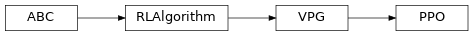Proximal Policy Optimization (PPO).

Parameters
• env_spec (EnvSpec) – Environment specification.

• policy (garage.torch.policies.Policy) – Policy.

• value_function (garage.torch.value_functions.ValueFunction) – The value function.

• policy_optimizer (garage.torch.optimizer.OptimizerWrapper) – Optimizer for policy.

• vf_optimizer (garage.torch.optimizer.OptimizerWrapper) – Optimizer for value function.

• lr_clip_range (float) – The limit on the likelihood ratio between policies.

• num_train_per_epoch (int) – Number of train_once calls per epoch.

• discount (float) – Discount.

• gae_lambda (float) – Lambda used for generalized advantage estimation.

• center_adv (bool) – Whether to rescale the advantages so that they have mean 0 and standard deviation 1.

• positive_adv (bool) – Whether to shift the advantages so that they are always positive. When used in conjunction with center_adv the advantages will be standardized before shifting.

• policy_ent_coeff (float) – The coefficient of the policy entropy. Setting it to zero would mean no entropy regularization.

• use_softplus_entropy (bool) – Whether to estimate the softmax distribution of the entropy to prevent the entropy from being negative.

• entropy_method (str) – A string from: ‘max’, ‘regularized’, ‘no_entropy’. The type of entropy method to use. ‘max’ adds the dense entropy to the reward for each time step. ‘regularized’ adds the mean entropy to the surrogate objective. See https://arxiv.org/abs/1805.00909 for more details.

property discount(self)

Discount factor used by the algorithm.

Returns

discount factor.

Return type

float

train(self, trainer)

Obtain samplers and start actual training for each epoch.

Parameters

trainer (Trainer) – Gives the algorithm the access to :method:~Trainer.step_epochs(), which provides services such as snapshotting and sampler control.

Returns

The average return in last epoch cycle.

Return type

float

class MAMLPPO(env, policy, value_function, task_sampler, inner_lr=_Default(0.1), outer_lr=0.001, lr_clip_range=0.5, discount=0.99, gae_lambda=1.0, center_adv=True, positive_adv=False, policy_ent_coeff=0.0, use_softplus_entropy=False, stop_entropy_gradient=False, entropy_method='no_entropy', meta_batch_size=20, num_grad_updates=1, meta_evaluator=None, evaluate_every_n_epochs=1)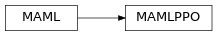Model-Agnostic Meta-Learning (MAML) applied to PPO.

Parameters
• env (Environment) – A multi-task environment.

• policy (garage.torch.policies.Policy) – Policy.

• value_function (garage.np.baselines.Baseline) – The value function.

• inner_lr (float) – Adaptation learning rate.

• outer_lr (float) – Meta policy learning rate.

• lr_clip_range (float) – The limit on the likelihood ratio between policies.

• discount (float) – Discount.

• gae_lambda (float) – Lambda used for generalized advantage estimation.

• center_adv (bool) – Whether to rescale the advantages so that they have mean 0 and standard deviation 1.

• positive_adv (bool) – Whether to shift the advantages so that they are always positive. When used in conjunction with center_adv the advantages will be standardized before shifting.

• policy_ent_coeff (float) – The coefficient of the policy entropy. Setting it to zero would mean no entropy regularization.

• use_softplus_entropy (bool) – Whether to estimate the softmax distribution of the entropy to prevent the entropy from being negative.

• entropy_method (str) – A string from: ‘max’, ‘regularized’, ‘no_entropy’. The type of entropy method to use. ‘max’ adds the dense entropy to the reward for each time step. ‘regularized’ adds the mean entropy to the surrogate objective. See https://arxiv.org/abs/1805.00909 for more details.

• meta_batch_size (int) – Number of tasks sampled per batch.

• meta_evaluator (garage.experiment.MetaEvaluator) – A meta evaluator for meta-testing. If None, don’t do meta-testing.

• evaluate_every_n_epochs (int) – Do meta-testing every this epochs.

train(self, trainer)

Obtain samples and start training for each epoch.

Parameters

trainer (Trainer) – Gives the algorithm access to :method:~Trainer.step_epochs(), which provides services such as snapshotting and sampler control.

Returns

The average return in last epoch cycle.

Return type

float

property policy(self)

Current policy of the inner algorithm.

Returns

Current policy of the inner

algorithm.

Return type

garage.torch.policies.Policy

get_exploration_policy(self)

Each time it is retrieved, this policy should only be evaluated in one task.

Returns

The policy used to obtain samples that are later used for

Return type

Policy

adapt_policy(self, exploration_policy, exploration_episodes)

Parameters
• exploration_policy (Policy) – A policy which was returned from get_exploration_policy(), and which generated exploration_episodes by interacting with an environment. The caller may not use this object after passing it into this method.

• exploration_episodes (EpisodeBatch) – Episodes with which to adapt, generated by exploration_policy exploring the environment.

Returns

exploration_episodes.

Return type

Policy

class TRPO(env_spec, policy, value_function, policy_optimizer=None, vf_optimizer=None, num_train_per_epoch=1, discount=0.99, gae_lambda=0.98, center_adv=True, positive_adv=False, policy_ent_coeff=0.0, use_softplus_entropy=False, stop_entropy_gradient=False, entropy_method='no_entropy')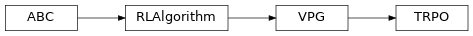Trust Region Policy Optimization (TRPO).

Parameters
• env_spec (EnvSpec) – Environment specification.

• policy (garage.torch.policies.Policy) – Policy.

• value_function (garage.torch.value_functions.ValueFunction) – The value function.

• policy_optimizer (garage.torch.optimizer.OptimizerWrapper) – Optimizer for policy.

• vf_optimizer (garage.torch.optimizer.OptimizerWrapper) – Optimizer for value function.

• num_train_per_epoch (int) – Number of train_once calls per epoch.

• discount (float) – Discount.

• gae_lambda (float) – Lambda used for generalized advantage estimation.

• center_adv (bool) – Whether to rescale the advantages so that they have mean 0 and standard deviation 1.

• positive_adv (bool) – Whether to shift the advantages so that they are always positive. When used in conjunction with center_adv the advantages will be standardized before shifting.

• policy_ent_coeff (float) – The coefficient of the policy entropy. Setting it to zero would mean no entropy regularization.

• use_softplus_entropy (bool) – Whether to estimate the softmax distribution of the entropy to prevent the entropy from being negative.

• entropy_method (str) – A string from: ‘max’, ‘regularized’, ‘no_entropy’. The type of entropy method to use. ‘max’ adds the dense entropy to the reward for each time step. ‘regularized’ adds the mean entropy to the surrogate objective. See https://arxiv.org/abs/1805.00909 for more details.

property discount(self)

Discount factor used by the algorithm.

Returns

discount factor.

Return type

float

train(self, trainer)

Obtain samplers and start actual training for each epoch.

Parameters

trainer (Trainer) – Gives the algorithm the access to :method:~Trainer.step_epochs(), which provides services such as snapshotting and sampler control.

Returns

The average return in last epoch cycle.

Return type

float

class MAMLTRPO(env, policy, value_function, task_sampler, inner_lr=_Default(0.01), outer_lr=0.001, max_kl_step=0.01, discount=0.99, gae_lambda=1, center_adv=True, positive_adv=False, policy_ent_coeff=0.0, use_softplus_entropy=False, stop_entropy_gradient=False, entropy_method='no_entropy', meta_batch_size=40, num_grad_updates=1, meta_evaluator=None, evaluate_every_n_epochs=1)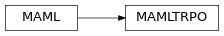Model-Agnostic Meta-Learning (MAML) applied to TRPO.

Parameters
• env (Environment) – A multi-task environment.

• policy (garage.torch.policies.Policy) – Policy.

• value_function (garage.np.baselines.Baseline) – The value function.

• inner_lr (float) – Adaptation learning rate.

• outer_lr (float) – Meta policy learning rate.

• max_kl_step (float) – The maximum KL divergence between old and new policies.

• discount (float) – Discount.

• gae_lambda (float) – Lambda used for generalized advantage estimation.

• center_adv (bool) – Whether to rescale the advantages so that they have mean 0 and standard deviation 1.

• positive_adv (bool) – Whether to shift the advantages so that they are always positive. When used in conjunction with center_adv the advantages will be standardized before shifting.

• policy_ent_coeff (float) – The coefficient of the policy entropy. Setting it to zero would mean no entropy regularization.

• use_softplus_entropy (bool) – Whether to estimate the softmax distribution of the entropy to prevent the entropy from being negative.

• entropy_method (str) – A string from: ‘max’, ‘regularized’, ‘no_entropy’. The type of entropy method to use. ‘max’ adds the dense entropy to the reward for each time step. ‘regularized’ adds the mean entropy to the surrogate objective. See https://arxiv.org/abs/1805.00909 for more details.

• meta_batch_size (int) – Number of tasks sampled per batch.

• meta_evaluator (garage.experiment.MetaEvaluator) – A meta evaluator for meta-testing. If None, don’t do meta-testing.

• evaluate_every_n_epochs (int) – Do meta-testing every this epochs.

train(self, trainer)

Obtain samples and start training for each epoch.

Parameters

trainer (Trainer) – Gives the algorithm access to :method:~Trainer.step_epochs(), which provides services such as snapshotting and sampler control.

Returns

The average return in last epoch cycle.

Return type

float

property policy(self)

Current policy of the inner algorithm.

Returns

Current policy of the inner

algorithm.

Return type

garage.torch.policies.Policy

get_exploration_policy(self)

Each time it is retrieved, this policy should only be evaluated in one task.

Returns

The policy used to obtain samples that are later used for

Return type

Policy

adapt_policy(self, exploration_policy, exploration_episodes)

Parameters
• exploration_policy (Policy) – A policy which was returned from get_exploration_policy(), and which generated exploration_episodes by interacting with an environment. The caller may not use this object after passing it into this method.

• exploration_episodes (EpisodeBatch) – Episodes with which to adapt, generated by exploration_policy exploring the environment.

Returns

exploration_episodes.

Return type

Policy

class SAC(env_spec, policy, qf1, qf2, replay_buffer, *, max_episode_length_eval=None, gradient_steps_per_itr, fixed_alpha=None, target_entropy=None, initial_log_entropy=0.0, discount=0.99, buffer_batch_size=64, min_buffer_size=int(10000.0), target_update_tau=0.005, policy_lr=0.0003, qf_lr=0.0003, reward_scale=1.0, optimizer=torch.optim.Adam, steps_per_epoch=1, num_evaluation_episodes=10, eval_env=None, use_deterministic_evaluation=True)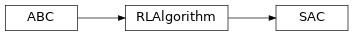A SAC Model in Torch.

Based on Soft Actor-Critic and Applications:

https://arxiv.org/abs/1812.05905

Soft Actor-Critic (SAC) is an algorithm which optimizes a stochastic policy in an off-policy way, forming a bridge between stochastic policy optimization and DDPG-style approaches. A central feature of SAC is entropy regularization. The policy is trained to maximize a trade-off between expected return and entropy, a measure of randomness in the policy. This has a close connection to the exploration-exploitation trade-off: increasing entropy results in more exploration, which can accelerate learning later on. It can also prevent the policy from prematurely converging to a bad local optimum.

Parameters
• policy (garage.torch.policy.Policy) – Policy/Actor/Agent that is being optimized by SAC.

• qf1 (garage.torch.q_function.ContinuousMLPQFunction) – QFunction/Critic used for actor/policy optimization. See Soft Actor-Critic and Applications.

• qf2 (garage.torch.q_function.ContinuousMLPQFunction) – QFunction/Critic used for actor/policy optimization. See Soft Actor-Critic and Applications.

• replay_buffer (ReplayBuffer) – Stores transitions that are previously collected by the sampler.

• env_spec (EnvSpec) – The env_spec attribute of the environment that the agent is being trained in.

• max_episode_length_eval (int or None) – Maximum length of episodes used for off-policy evaluation. If None, defaults to env_spec.max_episode_length.

• gradient_steps_per_itr (int) – Number of optimization steps that should

• gradient_steps_per_itr – Number of optimization steps that should occur before the training step is over and a new batch of transitions is collected by the sampler.

• fixed_alpha (float) – The entropy/temperature to be used if temperature is not supposed to be learned.

• target_entropy (float) – target entropy to be used during entropy/temperature optimization. If None, the default heuristic from Soft Actor-Critic Algorithms and Applications is used.

• initial_log_entropy (float) – initial entropy/temperature coefficient to be used if a fixed_alpha is not being used (fixed_alpha=None), and the entropy/temperature coefficient is being learned.

• discount (float) – Discount factor to be used during sampling and critic/q_function optimization.

• buffer_batch_size (int) – The number of transitions sampled from the replay buffer that are used during a single optimization step.

• min_buffer_size (int) – The minimum number of transitions that need to be in the replay buffer before training can begin.

• target_update_tau (float) – coefficient that controls the rate at which the target q_functions update over optimization iterations.

• policy_lr (float) – learning rate for policy optimizers.

• qf_lr (float) – learning rate for q_function optimizers.

• reward_scale (float) – reward scale. Changing this hyperparameter changes the effect that the reward from a transition will have during optimization.

• optimizer (torch.optim.Optimizer) – optimizer to be used for policy/actor, q_functions/critics, and temperature/entropy optimizations.

• steps_per_epoch (int) – Number of train_once calls per epoch.

• num_evaluation_episodes (int) – The number of evaluation episodes used for computing eval stats at the end of every epoch.

• eval_env (Environment) – environment used for collecting evaluation episodes. If None, a copy of the train env is used.

• use_deterministic_evaluation (bool) – True if the trained policy should be evaluated deterministically.

train(self, trainer)

Obtain samplers and start actual training for each epoch.

Parameters

trainer (Trainer) – Gives the algorithm the access to :method:~Trainer.step_epochs(), which provides services such as snapshotting and sampler control.

Returns

The average return in last epoch cycle.

Return type

float

train_once(self, itr=None, paths=None)

Complete 1 training iteration of SAC.

Parameters
• itr (int) – Iteration number. This argument is deprecated.

• paths (list[dict]) – A list of collected paths. This argument is deprecated.

Returns

loss from actor/policy network after optimization. torch.Tensor: loss from 1st q-function after optimization. torch.Tensor: loss from 2nd q-function after optimization.

Return type

torch.Tensor

optimize_policy(self, samples_data)

Optimize the policy q_functions, and temperature coefficient.

Parameters

samples_data (dict) – Transitions(S,A,R,S’) that are sampled from the replay buffer. It should have the keys ‘observation’, ‘action’, ‘reward’, ‘terminal’, and ‘next_observations’.

Note

samples_data’s entries should be torch.Tensor’s with the following shapes:

observation: $$(N, O^*)$$ action: $$(N, A^*)$$ reward: $$(N, 1)$$ terminal: $$(N, 1)$$ next_observation: $$(N, O^*)$$

Returns

loss from actor/policy network after optimization. torch.Tensor: loss from 1st q-function after optimization. torch.Tensor: loss from 2nd q-function after optimization.

Return type

torch.Tensor

property networks(self)

Return all the networks within the model.

Returns

A list of networks.

Return type

list

to(self, device=None)

Put all the networks within the model on device.

Parameters

device (str) – ID of GPU or CPU.

class MTSAC(policy, qf1, qf2, replay_buffer, env_spec, *, num_tasks, eval_env, gradient_steps_per_itr, max_episode_length_eval=None, fixed_alpha=None, target_entropy=None, initial_log_entropy=0.0, discount=0.99, buffer_batch_size=64, min_buffer_size=int(10000.0), target_update_tau=0.005, policy_lr=0.0003, qf_lr=0.0003, reward_scale=1.0, optimizer=torch.optim.Adam, steps_per_epoch=1, num_evaluation_episodes=5, use_deterministic_evaluation=True)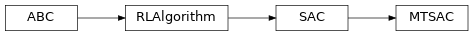A MTSAC Model in Torch.

This MTSAC implementation uses is the same as SAC except for a small change called “disentangled alphas”. Alpha is the entropy coefficient that is used to control exploration of the agent/policy. Disentangling alphas refers to having a separate alpha coefficients for every task learned by the policy. The alphas are accessed by using a the one-hot encoding of an id that is assigned to each task.

Parameters
• policy (garage.torch.policy.Policy) – Policy/Actor/Agent that is being optimized by SAC.

• qf1 (garage.torch.q_function.ContinuousMLPQFunction) – QFunction/Critic used for actor/policy optimization. See Soft Actor-Critic and Applications.

• qf2 (garage.torch.q_function.ContinuousMLPQFunction) – QFunction/Critic used for actor/policy optimization. See Soft Actor-Critic and Applications.

• replay_buffer (ReplayBuffer) – Stores transitions that are previously collected by the sampler.

• env_spec (EnvSpec) – The env_spec attribute of the environment that the agent is being trained in.

• max_episode_length_eval (int or None) – Maximum length of episodes used for off-policy evaluation. If None, defaults to max_episode_length.

• eval_env (Environment) – The environment used for collecting evaluation episodes.

• gradient_steps_per_itr (int) – Number of optimization steps that should occur before the training step is over and a new batch of transitions is collected by the sampler.

• fixed_alpha (float) – The entropy/temperature to be used if temperature is not supposed to be learned.

• target_entropy (float) – target entropy to be used during entropy/temperature optimization. If None, the default heuristic from Soft Actor-Critic Algorithms and Applications is used.

• initial_log_entropy (float) – initial entropy/temperature coefficient to be used if a fixed_alpha is not being used (fixed_alpha=None), and the entropy/temperature coefficient is being learned.

• discount (float) – The discount factor to be used during sampling and critic/q_function optimization.

• buffer_batch_size (int) – The number of transitions sampled from the replay buffer that are used during a single optimization step.

• min_buffer_size (int) – The minimum number of transitions that need to be in the replay buffer before training can begin.

• target_update_tau (float) – A coefficient that controls the rate at which the target q_functions update over optimization iterations.

• policy_lr (float) – Learning rate for policy optimizers.

• qf_lr (float) – Learning rate for q_function optimizers.

• reward_scale (float) – Reward multiplier. Changing this hyperparameter changes the effect that the reward from a transition will have during optimization.

• optimizer (torch.optim.Optimizer) – Optimizer to be used for policy/actor, q_functions/critics, and temperature/entropy optimizations.

• steps_per_epoch (int) – Number of train_once calls per epoch.

• num_evaluation_episodes (int) – The number of evaluation episodes used for computing eval stats at the end of every epoch.

• use_deterministic_evaluation (bool) – True if the trained policy should be evaluated deterministically.

to(self, device=None)

Put all the networks within the model on device.

Parameters

device (str) – ID of GPU or CPU.

train(self, trainer)

Obtain samplers and start actual training for each epoch.

Parameters

trainer (Trainer) – Gives the algorithm the access to :method:~Trainer.step_epochs(), which provides services such as snapshotting and sampler control.

Returns

The average return in last epoch cycle.

Return type

float

train_once(self, itr=None, paths=None)

Complete 1 training iteration of SAC.

Parameters
• itr (int) – Iteration number. This argument is deprecated.

• paths (list[dict]) – A list of collected paths. This argument is deprecated.

Returns

loss from actor/policy network after optimization. torch.Tensor: loss from 1st q-function after optimization. torch.Tensor: loss from 2nd q-function after optimization.

Return type

torch.Tensor

optimize_policy(self, samples_data)

Optimize the policy q_functions, and temperature coefficient.

Parameters

samples_data (dict) – Transitions(S,A,R,S’) that are sampled from the replay buffer. It should have the keys ‘observation’, ‘action’, ‘reward’, ‘terminal’, and ‘next_observations’.

Note

samples_data’s entries should be torch.Tensor’s with the following shapes:

observation: $$(N, O^*)$$ action: $$(N, A^*)$$ reward: $$(N, 1)$$ terminal: $$(N, 1)$$ next_observation: $$(N, O^*)$$

Returns

loss from actor/policy network after optimization. torch.Tensor: loss from 1st q-function after optimization. torch.Tensor: loss from 2nd q-function after optimization.

Return type

torch.Tensor

property networks(self)

Return all the networks within the model.

Returns

A list of networks.

Return type

list

class PEARL(env, inner_policy, qf, vf, *, num_train_tasks, num_test_tasks=None, latent_dim, encoder_hidden_sizes, test_env_sampler, policy_class=ContextConditionedPolicy, encoder_class=MLPEncoder, policy_lr=0.0003, qf_lr=0.0003, vf_lr=0.0003, context_lr=0.0003, policy_mean_reg_coeff=0.001, policy_std_reg_coeff=0.001, policy_pre_activation_coeff=0.0, soft_target_tau=0.005, kl_lambda=0.1, optimizer_class=torch.optim.Adam, use_information_bottleneck=True, use_next_obs_in_context=False, meta_batch_size=64, num_steps_per_epoch=1000, num_initial_steps=100, num_tasks_sample=100, num_steps_prior=100, num_steps_posterior=0, num_extra_rl_steps_posterior=100, batch_size=1024, embedding_batch_size=1024, embedding_mini_batch_size=1024, discount=0.99, replay_buffer_size=1000000, reward_scale=1, update_post_train=1)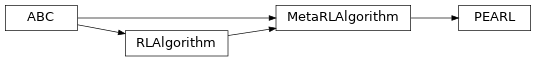A PEARL model based on https://arxiv.org/abs/1903.08254.

PEARL, which stands for Probablistic Embeddings for Actor-Critic Reinforcement Learning, is an off-policy meta-RL algorithm. It is built on top of SAC using two Q-functions and a value function with an addition of an inference network that estimates the posterior $$q(z \| c)$$. The policy is conditioned on the latent variable Z in order to adpat its behavior to specific tasks.

Parameters
• env (list[Environment]) – Batch of sampled environment updates( EnvUpdate), which, when invoked on environments, will configure them with new tasks.

• policy_class (type) – Class implementing :pyclass:~ContextConditionedPolicy

• encoder_class (garage.torch.embeddings.ContextEncoder) – Encoder class for the encoder in context-conditioned policy.

• inner_policy (garage.torch.policies.Policy) – Policy.

• qf (torch.nn.Module) – Q-function.

• vf (torch.nn.Module) – Value function.

• latent_dim (int) – Size of latent context vector.

• encoder_hidden_sizes (list[int]) – Output dimension of dense layer(s) of the context encoder.

• policy_lr (float) – Policy learning rate.

• qf_lr (float) – Q-function learning rate.

• vf_lr (float) – Value function learning rate.

• context_lr (float) – Inference network learning rate.

• policy_mean_reg_coeff (float) – Policy mean regulation weight.

• policy_std_reg_coeff (float) – Policy std regulation weight.

• policy_pre_activation_coeff (float) – Policy pre-activation weight.

• soft_target_tau (float) – Interpolation parameter for doing the soft target update.

• kl_lambda (float) – KL lambda value.

• optimizer_class (type) – Type of optimizer for training networks.

• use_information_bottleneck (bool) – False means latent context is deterministic.

• use_next_obs_in_context (bool) – Whether or not to use next observation in distinguishing between tasks.

• meta_batch_size (int) – Meta batch size.

• num_steps_per_epoch (int) – Number of iterations per epoch.

• num_initial_steps (int) – Number of transitions obtained per task before training.

• num_tasks_sample (int) – Number of random tasks to obtain data for each iteration.

• num_steps_prior (int) – Number of transitions to obtain per task with z ~ prior.

• num_steps_posterior (int) – Number of transitions to obtain per task with z ~ posterior.

• num_extra_rl_steps_posterior (int) – Number of additional transitions to obtain per task with z ~ posterior that are only used to train the policy and NOT the encoder.

• batch_size (int) – Number of transitions in RL batch.

• embedding_batch_size (int) – Number of transitions in context batch.

• embedding_mini_batch_size (int) – Number of transitions in mini context batch; should be same as embedding_batch_size for non-recurrent encoder.

• discount (float) – RL discount factor.

• replay_buffer_size (int) – Maximum samples in replay buffer.

• reward_scale (int) – Reward scale.

• update_post_train (int) – How often to resample context when obtaining data during training (in episodes).

train(self, trainer)

Obtain samples, train, and evaluate for each epoch.

Parameters

trainer (Trainer) – Gives the algorithm the access to :method:Trainer..step_epochs(), which provides services such as snapshotting and sampler control.

property policy(self)

Return all the policy within the model.

Returns

Policy within the model.

Return type

garage.torch.policies.Policy

property networks(self)

Return all the networks within the model.

Returns

A list of networks.

Return type

list

get_exploration_policy(self)

Each time it is retrieved, this policy should only be evaluated in one task.

Returns

The policy used to obtain samples that are later used for

Return type

Policy

adapt_policy(self, exploration_policy, exploration_episodes)

Parameters
• exploration_policy (Policy) – A policy which was returned from get_exploration_policy(), and which generated exploration_episodes by interacting with an environment. The caller may not use this object after passing it into this method.

• exploration_episodes (EpisodeBatch) – Episodes to which to adapt, generated by exploration_policy exploring the environment.

Returns

exploration_episodes.

Return type

Policy

to(self, device=None)

Put all the networks within the model on device.

Parameters

device (str) – ID of GPU or CPU.

classmethod augment_env_spec(cls, env_spec, latent_dim)

Augment environment by a size of latent dimension.

Parameters
• env_spec (EnvSpec) – Environment specs to be augmented.

• latent_dim (int) – Latent dimension.

Returns

Augmented environment specs.

Return type

EnvSpec

classmethod get_env_spec`(cls, env_spec, latent_dim, module)

Get environment specs of encoder with latent dimension.

Parameters
• env_spec (EnvSpec) – Environment specification.

• latent_dim (int) – Latent dimension.

• module (str) – Module to get environment specs for.

Returns

Module environment specs with latent dimension.

Return type

InOutSpec